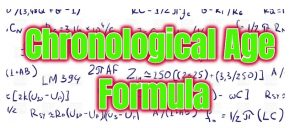# Chronological Age Formula

Chronological Age Formula: Chronological age depends on your birthday. Without a birth date, you can not calculate your chronological age. So if you want to calculate your chronological age then this article will be helpful for you Because I am explaining some Chronological age formula, which can help you.

firstly we try to calculate understand the chronological age. And we will try to know what it depends on. so let’s start.

## Chronological Age

Chronological age is the age which defines that How many old you are. The chronological age depends on your date of birth. And with the help of date of birth, you can calculate your chronological age, and you can find out how many years you lived.

Basically chronological age is your original age. Everyone is born on a fixed date. Gradually it grows. So all the years that he lives, the whole age is called his chronological age.

So I hope you understand. And now we will know that what is Chronological age formula by which you can calculate your chronological age.## Chronological Age Formula

This is very simple and you can calculate your chronological age easily. I want to say again that chronological age depends on your birth date. Without your birth date, you can not calculate your chronological age. So firstly you know your date of birth.

• Then divide your date of birth into the month, date and year.
• And then know the today date and divide into date, month year.
• Now reduce your date of birth from today’s date.

So in this way you can calculate your chronological age without any calculator.

For Example

If your Date of Birth is 26 May 1995 and today date is 29 August 2019.

So firstly divided your date of birth and today’s date into the month, Date and year.

Date of Birth = 5, 26 , 1995

Today’s date = 8, 29, 2019

Then Chronological Age = 24 year, 3 month and 3 day.

 Date of Birth 5 26 1995 Today’s Date 8 29 2019 Chronological Age 3 month 3 day 24 year

For Example

If your date of birth is 22 February 1997 and Today’s date is 30 August 2019.

Date of Birth = 2, 22, 1997

Today Date = 8, 30, 2019

Chronological Age = 6 Month, 8 days, 22 year

## Another Chronological Age Formula

You can calculate your age with the help of Intelligence Quotient (IQ). So if mental age and Chronological age are given to us, then there is a theoretical formula to calculate the IQ of a person.

 IQ =(Mental age / Chronological age)100

For Example

If a child has a mental age of 5 years and IQ is 125 then

 Formula IQ = (Mental Age / Chronological Age) 100 Chronological Age = (Mental Age / IQ) 100

Chronological Age = ( 5 / 125) 100

Chronological Age =  4

### Final Word

So, friend, this is the information related to Chronological Age Formula. And I hope you understand and know you can calculate your chronological age. So if you have any suggestion related to chronological Age Formula, then you can comment.